# Matplotlib Surface Plot – Tutorial for Beginners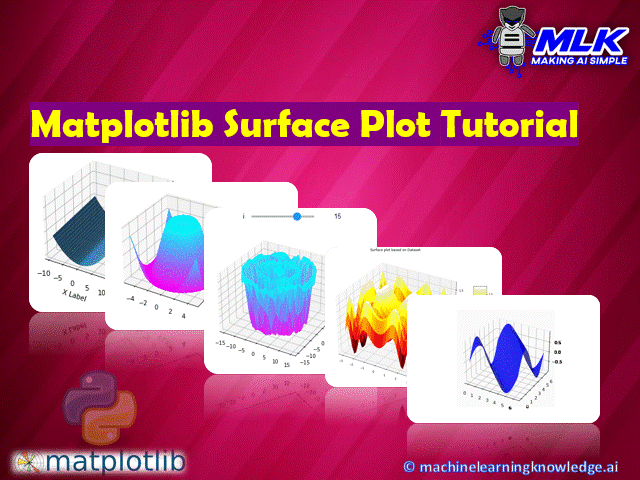## Introduction

In this article, we will go through the tutorial for the matplotlib surface plot for your data science and machine learning project. We will discuss varieties of 2D and 3D surface charts with multiple examples for better understanding. Throughout this tutorial, we will learn about the details that should be taken care of while working with these 2D and 3D surface charts in Matplotlib.

### Importing Matplotlib Library

Before beginning with this matplotlib bar plot tutorial, we’ll need Matplotlib and other relevant libraries for our examples.

In :
```import matplotlib.pyplot as plt
import numpy as np
import pandas as pd```

### Example 1 : Simple Matplotlib Surface Plot in 3D

The first example of surface plot shows how a simple 3D surface plot can be built. Initially, data is generated with the help of arange function. The data is arranged over a meshgrid and then plot_surface is called for plotting a surface plot.

In this function, the data for three dimensions is provided which helps in plotting.

In :
```from mpl_toolkits.mplot3d import Axes3D
import random

def fun(x, y):
return x**2 + y

fig = plt.figure()
x = y = np.arange(-9.5, 15.0, 0.15)
X, Y = np.meshgrid(x, y)
zs = np.array(fun(np.ravel(X), np.ravel(Y)))
Z = zs.reshape(X.shape)

ax.plot_surface(X, Y, Z)

ax.set_xlabel('X Label')
ax.set_ylabel('Y Label')
ax.set_zlabel('Z Label')

plt.show()
```
Output: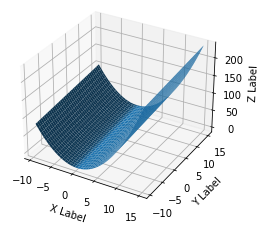### Example 2 : 3D Surface Plot in Matplotlib with Colorbar

The 2nd example will teach you how you can build a 3D Surface Plot. First, we import some mandatory functions of the matplotlib library. With the help of arange function, we can generate random data for the three dimensions of the plot.

Again the plot_surface is called for plotting 3D Surface plot. You can also set the range for all the axes. To make the plot more informative, we can build a colorbar by using colorbar function of matplotlib.

In :
```from mpl_toolkits.mplot3d import Axes3D
from matplotlib import cm
from matplotlib.ticker import LinearLocator, FormatStrFormatter

fig = plt.figure()
ax = fig.gca(projection='3d')

# Generating data.
X = np.arange(-5, 5, 0.25)
Y = np.arange(-5, 5, 0.25)
X, Y = np.meshgrid(X, Y)
R = np.sqrt(X**2 + Y**2)
Z = np.sin(R)

# Plot the surface.
surf = ax.plot_surface(X, Y, Z, cmap=cm.cool_r,
linewidth=0, antialiased=False)

# Customize the z axis.
ax.set_zlim(-1.01, 1.01)
ax.zaxis.set_major_formatter(FormatStrFormatter('%.02f'))

# Add a color bar which maps values to colors.
fig.colorbar(surf, shrink=0.5, aspect=7)

plt.show()
```
Output: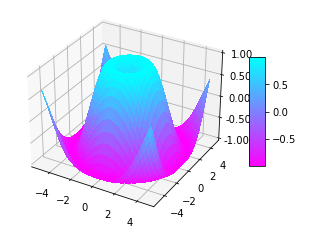### Example 3 : Interactive Surface Plot with Matplotlib

In this example, we will see how to make an interactive surface plot with matplotlib by adding a slider. The majority of the work is performed with the help of functions imported from Matplotlib.

To plot this type of surface plot, we call plot_trisurf function. We pass the data for all 3 dimensions, color, and other relevant details.

For making surface plot interactive, we are calling the interactive function of ipywidgets library. This interactive function is provided with the plot function, this is used for plotting.

In :
```from __future__ import print_function
from ipywidgets import interact, interactive, fixed, interact_manual
import ipywidgets as widgets
from IPython.display import Image

from mpl_toolkits.mplot3d import Axes3D
from mpl_toolkits import mplot3d

def f(x, y):
return np.sin(np.sqrt(x ** 2 + y ** 2))

def plot(i):

fig = plt.figure()
ax = plt.axes(projection='3d')

theta = 2 * np.pi * np.random.random(1000)
r = i * np.random.random(1000)
x = np.ravel(r * np.sin(theta))
y = np.ravel(r * np.cos(theta))
z = f(x, y)

ax.plot_trisurf(x, y, z, cmap='cool_r', edgecolor='none')
fig.tight_layout()

interactive_plot = interactive(plot, i=(2, 20))
interactive_plot
```
Output: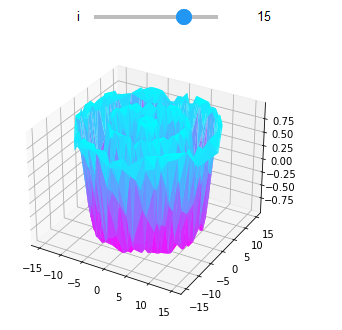Example 4 : Surface Plot based on Dataset

This example shows how to use the available dataset for plotting an intuitive surface plot. Here random dataset is generated and then assigned to all the three variables, representing the three dimensions. For plotting the figure, we use figure function. The final surface plot has a colorbar along with designated colors.

In :
```# Creating dataset
x = np.outer(np.linspace(-3, 3, 32), np.ones(32))
y = x.copy().T # transpose
z = (np.sin(x **2) + np.cos(y **2) )

# Creating figyre
fig = plt.figure(figsize =(14, 9))
ax = plt.axes(projection ='3d')

# Creating color map
my_cmap = plt.get_cmap('hot')

# Creating plot
surf = ax.plot_surface(x, y, z, cmap = my_cmap, edgecolor ='none')

fig.colorbar(surf, ax = ax, shrink = 0.5, aspect = 5)

ax.set_title('Surface plot based on Dataset')

# show plot
plt.show()
```
Output: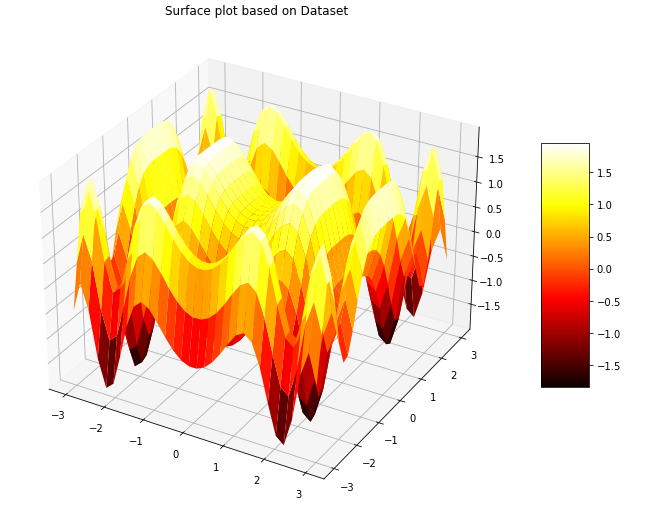### Example 5 : Animated Surface Plot

For this example, we have imported the animation function from the matplotlib library. Here we learn how animation can be added to a surface plot.

The feature of animation is added with the help of FuncAnimation function that is contained in the animation function.

To save the results for future reference, the movement can be stored in a .gif file.

In :
```import matplotlib.animation as animation
from mpl_toolkits.mplot3d import Axes3D

def data(i, z, line):
z = np.sin(x+y+i)
ax.clear()
line = ax.plot_surface(x, y, z,color= 'b')
return line,

n = 2.*np.pi
fig = plt.figure()

x = np.linspace(0,n,100)
y = np.linspace(0,n,100)
x,y = np.meshgrid(x,y)
z = np.sin(x+y)
line = ax.plot_surface(x, y, z,color= 'r')

ani = animation.FuncAnimation(fig, data, fargs=(z, line), interval=30, blit=False)
ani.save('surface.gif', dpi=80, writer='imagemagick', fps=24)

plt.show()
```
Output: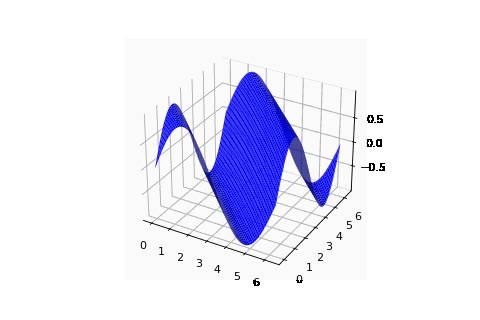## Conclusion

We have reached to the end of this tutorial focusing on building 2D and 3D surface plot with matplotlib, in this we learned about different types of surface plots that can be build using matplotlib. The plots covered talk about the intricate details that should be taken care of while building a variety of useful 2D and 3D plots. We also looked at how we can play around with the parameters for producing a combination of different surface plots.

Reference –  Matplotlib Documentation